# Divisibility of integers, divisibility rules

Definition: an Integer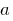divided by an integer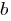, if there is such a number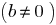that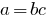.

The numberis called a divisor of the number, and the numberis a multiple of the number.

## Properties of divisibility

1. If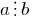and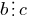, then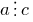.
2. Ifand,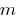and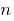is any integer, then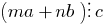.
3. Ifand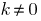that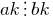.
4. Ifand, thenand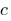vsampler number.

## Divisibility rules

### Divisibility of number 2

The last digit of the number is divisible by 2 (even).

An integerthat is divisible by 2 is called even, and it can be represented in the form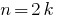where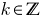.

An integerthat is not divisible by 2 are called oddand can be represented in the form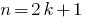where.

### Divisibility of numbers by 3

The sum of the digits is divisible by 3.

For example, the number 822. It does not contain any triples, but the sum of its figures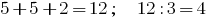is divisible by 3 evenly, therefore the divisibility rules 822 is divisible by 3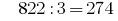.

### Divisibility rule of 10

The number ends with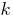zeros.

### Divisibility of the number 4

The number expressed by the last two digits of a given number is divisible by 4.

For example, the number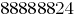is large enough for division students in the 7th grade.

However potrebno only need to check divisibility by 4 last two digits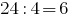, we can conclude that 88888824 has a divisor of four.

### Divisibility of the number 7

Rule of divisibility by 7 of large numbers. Mentally break the number into blocks of three digits, starting from the last digit. According to the rules, if the difference of the sum of blocks, standing in the even places and the sum of blocks, standing at odd places, is divided by 7, the number is divisible by 7.

For example,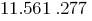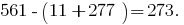Check 273 the rule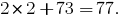This number is "beautiful" is divided into 7. So to check divisibility of a number by 7 and solve the example have the possibility for multiple rules. Each of them has for a number of numbers certain advantages over the other, so choose which way is more intuitive and faster.

### Divisibility of the number 5

The last digit of the number is 0 or 5.

### Divisibility of the number 8

The number expressed by the last three digits of the given number is divisible by 8.

### Divisibility of numbers by 9

The sum of digits of number divisible by 9.

### Divisibility of numbers by 11

The difference between the sum of the digits standing in the odd places (counting right to left), and the sum of the digits standing in the even positions (counting from right to left) is divisible by 11.

Tags:
Chapter:
Versions in other languages:
Share with friends: